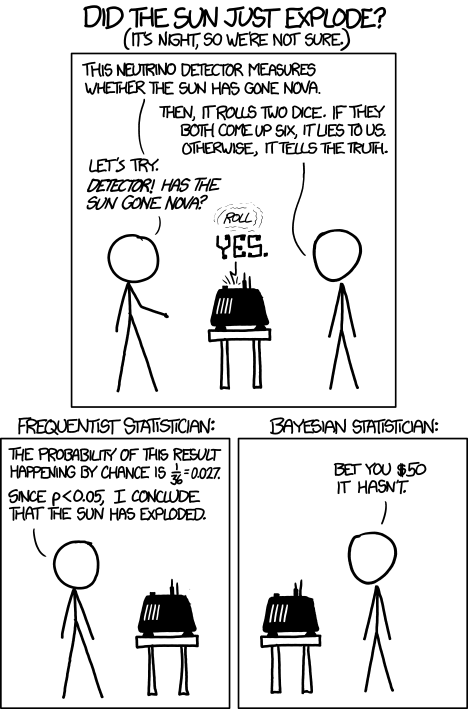# XKCD: Frequentist vs. Bayesian StatisticsTwo approaches to problems in the world of statistics and machine learning are that of frequentist and Bayesian statistics. This comic from XKCD illustrates a difference between the two viewpoints.We have a neutrino detector that measures whether the sun has gone nova. The detector is not perfect; 1/36 times, the detector incorrectly indicates whether or not the sun has gone nova. [The probability of rolling two fair die as both 6 is 1/6 * 1/6 = 1/36.]

Next, we push the button on the detector to try it, and it indicates that the sun has gone nova. Has the sun indeed exploded? [The sun is shining on the other side of the earth, and there is a time lag for the light from the sun to reach us.]

The frequentist might make the following point. The probability that the detector would lie to us is 1/36. That is quite small…

The Bayesian statistician cannot argue with this point. However, and this is the distinction between the two mindsets, the Bayesian statistician would take his or her prior knowledge into account that the probability of the sun exploding today is very small, so most likely the detector is lying, in spite of the fact that the detector is quite unlikely to lie.

Let’s put this into some formal mathematics. Define two events:

$E$: the sun has Exploded

$D$: the neutrino Detector has detected an explosion.

We know that the probability that the detector has detected an explosion given that there was not actually an explosion is $P(D | ~E) = 1/36$. The quantity that we’re interested in for making this bet is the probability that there was actually an explosion given the data that the detector has detected an explosion, $P(E | D)$. A fancy name for this probability is the posterior probability.

To get the posterior $P(E | D)$, we need to use Bayes’ theorem:

$$P(E | D) = \dfrac{P(D | E) P(E)}{P(D)}.$$

The term $P(D | E)$ is called the likelihood; this is the probability of observing the data given the event. Frequentists focus on the likelihood when determining whether or not an event has occurred. In this case,

$$P(D | E) = 1 - P(~D | E) = 1 - 1/36 = 35/36.$$

The likelihood is quite high, so the frequentist might conclude that the sun indeed exploded.

However, of course, Bayes’ theorem tells us that there is another contribution to the story: the term $P(E)$, which is called the prior. This is simply the probability that the sun has exploded. We know that this is indeed very small, and the Bayesian statistician would inject this “prior knowledge” into the problem, hence taking the prior probability $P(E)$ into account in determining $P(E | D)$.

The probability of the detector detecting an explosion $P(D)$ is somewhat redundant since we can write it in terms of other quantities:

$$P(D) = P(D | E) P(E) + [1 - P(D | E)] [1 - P(E)].$$

Note that this term goes to 1/36 as $P(E)$ gets small and is 35/36 if $P(E) = 1$ (i.e. if the sun exploded with certainty) .

Thus, we should write the probability that the sun actually exploded, given our evidence that the neutrino detector went off,

$$P(E | D) = \dfrac{ \frac{35}{36} P(E)}{\frac{34}{36} P(E) + \frac{1}{36}}.$$

At small prior probabilities $P(E)$, this looks like

$$P(E | D) ~ 35 P(E).$$

The Bayesian statistician knows that the astronomically small prior $P(E)$ overwhelms the high likelihood $P(D | E)$.

In this problem, we clearly have a reason to inject our belief/prior knowledge that $P(E)$ is very small, so it is very easy to agree with the Bayesian statistician. However, in many cases, it is difficult or unjustified to assume prior knowledge $P(E)$.

This bet is quite moot anyway. Consider the loss function: if the Bayesian statistician is correct, you lose $50. If the Bayesian statistician is incorrect, the world will end and you probably won’t have a chance to spend the$50.